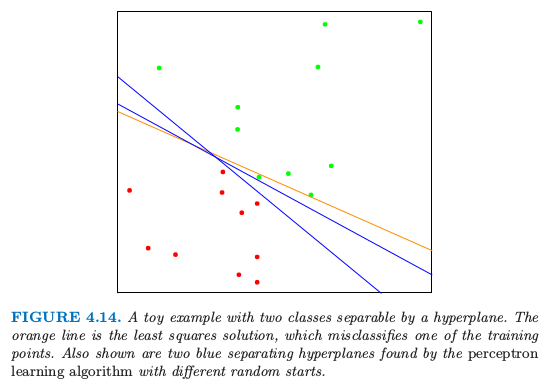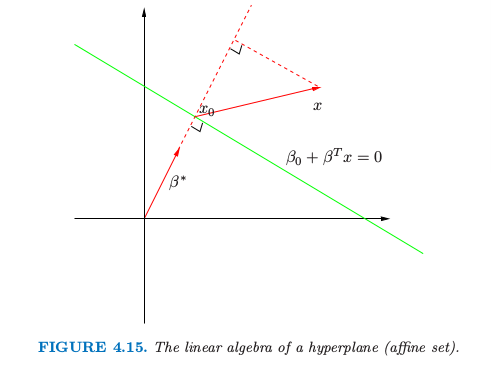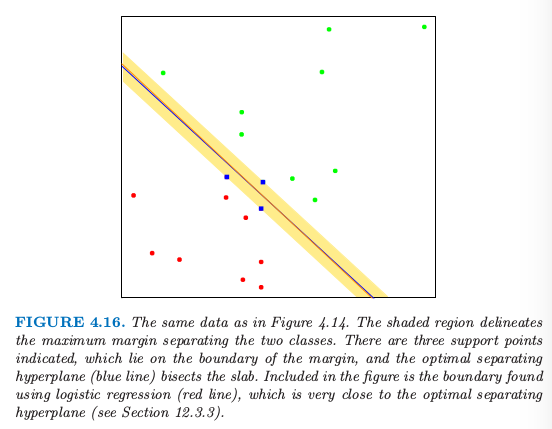4.5 分离超平面¶weiya 注：Ex. 4.2

1. 对于 $L$ 中的两点 $x_1$ 和 $x_2$，$\beta^T(x_1-x_2)=0$，因此 $L$ 表面的法向量为 $\beta^*=\beta/\Vert\beta\Vert$.
2. 对于 $L$ 的任意点，$\beta^Tx_0=-\beta_0$.
3. 任意点 $x$ 到 $L$ 的符号距离为 因此 $f(x)$ 与 $x$ 到由 $f(x)=0$ 定义的超平面符号距离成比例．4.5.1 Rosenblatt 感知器学习算法¶

Ripley (1996)2 总结了该算法有以下一些问题：

• 当数据是线性可分时，有许多解，且解基于初始值的设定．
• “有限”步可以非常大．差异越小，需要花的时间就越久．
• 当数据不是线性可分时，算法不会收敛，而且会形成循环．循环可以很长因此不容易检测．

4.5.2 最优分离超平面¶

（重新定义了 $\beta_0$）或者等价于

weiya 注：对偶问题

weiya 注：KKT条件

• 如果 $\alpha_i\gt 0$，则 $y_i(x_i^T\beta+\beta_0)=1$，或者换句话说，$x_i$ 在 slab 的边界上；
• 如果 $y_i(x_i^T\beta+\beta_0)>1$，$x_i$ 不在平板的边界上，而且 $\alpha_i=0$．1. Rosenblatt, F. (1958). The perceptron: a probabilistic model for information storage and organization in the brain, Psychological Review 65: 386–408.

2. Ripley, B. D. (1996). Pattern Recognition and Neural Networks, Cambridge University Press.

3. Vapnik, V. (1996). The Nature of Statistical Learning Theory, Springer, New York.